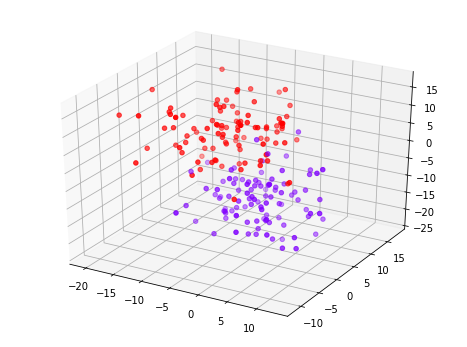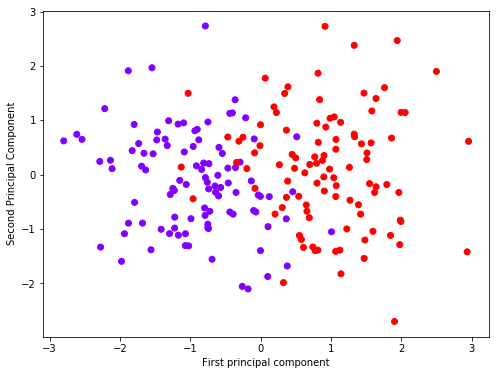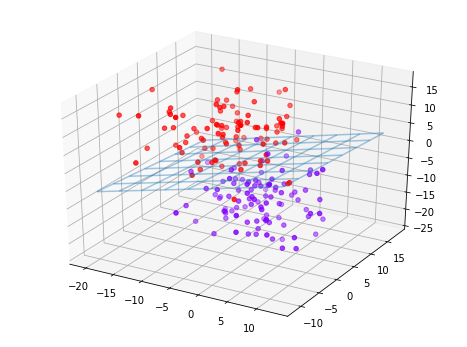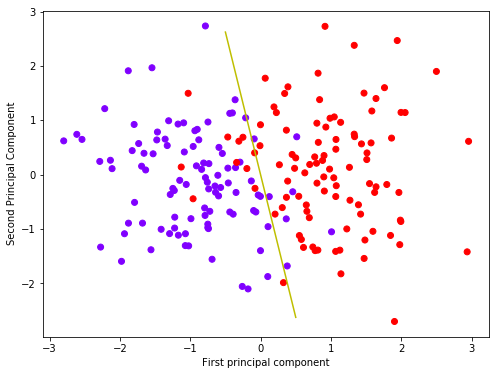# Principal Component Analysis (PCA) Part 1¶

Elan Ding
Modified: June 8, 2018

In this post, we will discuss PCA in a practical perspectives.

## What is PCA?¶

PCA is a technique used to reduce the number of features, making it easier to visualize a high-dimensional dataset. By using the first two principle components, we can visualize a high-dimensional dataset in a new two-dimensional space. In exchange for the visulization, we lose some interpretability.

## Generating data¶

First let's import some libraries. The mplot3d is used for creating 3d plots.

In :
import matplotlib.pyplot as plt
from mpl_toolkits import mplot3d
import pandas as pd
import numpy as np
import seaborn as sns
%matplotlib inline


Now we are ready to generate a dataset using sklearn's make_blobs. Here we are generaing a dataset with three features and binary labels. We can think of each data point as a patient who is either positive of negative of a given disease.

In :
from sklearn.datasets import make_blobs
data = make_blobs(n_samples=200, n_features=3,
centers=2, cluster_std=5,random_state=101)

In :
fig = plt.figure(figsize=(8,6))
ax = plt.axes(projection='3d')
ax.scatter(data[:,0], data[:,1], data[:,2], c=data, cmap='rainbow')

Out:
<mpl_toolkits.mplot3d.art3d.Path3DCollection at 0x1a1cadcb38>As you can see there are two clusters of data. The overlapping is due to standard deviation of the clusters since I set cluster_std = 5 in my code above.

In :
df = pd.DataFrame(data, columns=['x', 'y', 'z'])

Out:
x y z
0 1.936270 6.449972 -7.781597
1 10.475693 -3.818440 -15.962927
2 10.748065 -0.469242 -8.278834
3 3.533531 3.873881 6.053832
4 2.972040 -1.531651 -8.487039

## PCA using Python¶

In order for PCA to perform well, we need every feature to have an unit variance.

In :
from sklearn.preprocessing import StandardScaler
scaler = StandardScaler()
scaler.fit(df)
scaled_data = scaler.transform(df)


Next, we fit scaled_data into sklearn's PCA class.

In :
from sklearn.decomposition import PCA
pca = PCA(n_components=2)
pca.fit(scaled_data)
x_pca = pca.transform(scaled_data)


After using the transform method, we have reduced the number of features to 2.

In :
print(scaled_data.shape)
print(x_pca.shape)

(200, 3)
(200, 2)


## Visualizing PCA¶

We plot the first and second principal components colored by the label.

In :
plt.figure(figsize=(8,6))
plt.scatter(x_pca[:,0],x_pca[:,1],c=data,cmap='rainbow')
plt.xlabel('First principal component')
plt.ylabel('Second Principal Component')

Out:
Text(0,0.5,'Second Principal Component')The principle components are simply linear combinations of the original feature vectors. The coefficients of the linear combination can be found by the following.

In :
pca.components_

Out:
array([[-0.68376876,  0.25721576,  0.68286188],
[-0.17804995, -0.96633893,  0.18570756]])

Let $x,y,z$ be the original features. The array above means that approximately,

\begin{aligned} \text{First Principal Component} &= -0.68x + 0.26y + 0.68z \\ \text{Second Principal Component} &= -0.18x - 0.97y + 0.19z \end{aligned}

## Compare Decision Boundaries¶

Using a machine learning technique called linear discriminant analysis, we can find the so called Bayes' decision boundary, which minimizes the total error in our classification. This can be found using sklearn easily.

In :
from sklearn.discriminant_analysis import LinearDiscriminantAnalysis
clf2 = LinearDiscriminantAnalysis()
clf2.fit(data, data)
print(clf2.coef_)
print(clf2.intercept_)

[[-0.20763429  0.06824359  0.52744419]]
[0.14878286]

In :
fig = plt.figure(figsize=(8,6))
ax = plt.axes(projection='3d')
ax.scatter(data[:,0], data[:,1], data[:,2], c=data, cmap='rainbow')

xx, yy = np.meshgrid(range(-20,15,4), range(-10,20,4))
z = (0.20763429/0.52744419)*xx - (0.06824359/0.52744419)*yy + 0.14878286
ax.plot_wireframe(xx, yy, z, alpha=0.4)

Out:
<mpl_toolkits.mplot3d.art3d.Line3DCollection at 0x1a1d7a35f8>The surface above is the Bayes' decision boundary. It is a linear function. Given that the two classes have the same covariance matrix, it is the best we can do in terms of minimizing total classification error.

Here comes the advantage of PCA. What if our original data has more than 3 dimensions? It is impossible to directly visualize something hither than dimension 3, so by reducing the dimension down to 2 using PCA, we get a nice visualization of the classification problem.

In :
clf = LinearDiscriminantAnalysis()
clf.fit(x_pca, data)
print(clf.coef_)
print(clf.intercept_)

[[3.4014186 0.6482249]]
[4.4408921e-16]

In :
plt.figure(figsize=(8,6))
plt.scatter(x_pca[:,0],x_pca[:,1],c=data,cmap='rainbow')
plt.xlabel('First principal component')
plt.ylabel('Second Principal Component')
x = np.linspace(-.5, .5, 100)
y = -(3.4014186/0.6482249)*x
plt.plot(x,y, c='y')

Out:
[<matplotlib.lines.Line2D at 0x1a1d7e2630>]The yellow line is the Bayes' decision boundary in terms of the principal components.

That was fun, right? In the next post, we will discuss the theoretical background to better understand why PCA works.